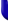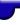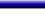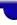Lighthouse3d.com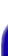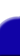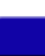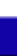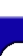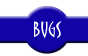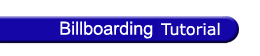Billboarding Tutorial Index Cheating - Fast and Easy Cheating - Faster but not so Easy True Billboards# Billboarding Tutorial

## Cheating - Cylindrical Billboards

As mentioned in the previous section, the modelview matrix contains the transformations required to perform the change of coordinates between local coordinates and world coordinates. It has been shown that by replacing the top 3x3 submatrix with the identity matrix a cheating version of spherical billboarding can be obtained. But what about Cylindrical billboarding?

Let us analyze in depth what is actually the structure of the top 3X3 submatrix. This matrix contains 3 columns, and each has a special meaning. The first is the right vector, the second column is the up vector and the 3rd represents the lookAt vector. These vectors are relative to the cameras orientation. Setting the top 3x3 submatrix to the identity matrix effectively causes the camera's coordinate system to be aligned with the worlds coordinate system.

In order to achieve a (cheating) Cylindrical Billboard, it is sufficient to align the right and lookAt vectors, leaving the up vector unchanged. The difference between this version and the spherical one, presented in the previous section, is that when the camera looks up or down the billboard won't move. The billboard will only be rotated when the camera looks right or left.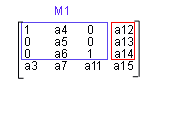This type of billboards can be applied to trees. Trees don't bend backwards and forwards as the camera looks down or up because the up vector is fixed, so it doesn't make much sense to use a spherical billboard. Beware that the illusion is broken when you fly over the tree. In this case you'll see your tree getting thinner and thinner and all is lost. However if you're application keeps you on the terrain then cylindrical billboarding is a good option.

The code for cheating cylindrical billboards is almost identical to the spherical version.

```    ```
float modelview;
int i,j;

// save the current modelview matrix
glPushMatrix();

// get the current modelview matrix
glGetFloatv(GL_MODELVIEW_MATRIX , modelview);

// The only difference now is that
// the i variable will jump over the
// up vector, 2nd column in OpenGL convention

for( i=0; i<3; i+=2 )
for( j=0; j<3; j++ ) {
if ( i==j )
modelview[i*4+j] = 1.0;
else
modelview[i*4+j] = 0.0;
}

// set the modelview matrix with the
// up vector unchanged

drawObject();

// restores the modelview matrix
glPopMatrix();
```
```

As we did before, the above code can be divided in two functions, besides the rendering function, drawObject. The first function will setup the modelview matrix, and the second will restore the current matrix.

```    ```
void billboardCheatCylindricalBegin() {

float modelview;
int i,j;

// save the current modelview matrix
glPushMatrix();

// get the current modelview matrix
glGetFloatv(GL_MODELVIEW_MATRIX , modelview);

for( i=0; i<3; i+=2 )
for( j=0; j<3; j++ ) {
if ( i==j )
modelview[i*4+j] = 1.0;
else
modelview[i*4+j] = 0.0;
}

// set the modelview matrix
}

void billboardEnd() {

// restore the previously
// stored modelview matrix
glPopMatrix();
}
```
```

The source code for rendering a billboard object becomes:

```    ```
billboardCheatCylindricalBegin();
drawObject();
billboardEnd();
```
```

Again, beware with scaling operations. Scaling in the X and Y axis will be undone using this approach, but scaling in the Z axis will persist. the best option is to scale after you call billboardCheatCylindricalBegin().